Question

Circuits

A generic Bode plot for this amplifier configuration is also shown. We want to operate the amplifier at max gain between frequencies f3 and f4, which are about 15 Hz and 5 kHz respectively.

1. Discuss/comment on the usefulness of the coupling capacitor C1 which is connected to the drain.

2. Does the maxgain change if C¡ is not there (or shorted at all frequencies of interest)?

3. Complete the amplifier design, i.e. determine all resistor and capacitor values.

4. Determine fi and f2. You can assume that Rsi = 200 Q. [Hint: these two frequencies need not be distinct if the desired bandwidth for maximum gain is not affected]

5. Determine the maximum gain, A in the plot shown. Also determine B in the plot if you must design the circuit to match the Bode plot to the one shown.

6. Pick a value for C1 such that it can be neglected in your analytical calculations but does not significantly affect the Bode plot and justify your choice.

7. The amplifier shown is to be used as a pre-amplifier, i.e. vo is to become vị to another amplifier circuit, that is to be constructed with a MOSFET with the same parameters. The second amplifier should have about the same gain as the one shown. Discuss which elements of the circuit shown can be omitted in the second circuit and justify your answer. Discuss the feasibility of this composite design.Verified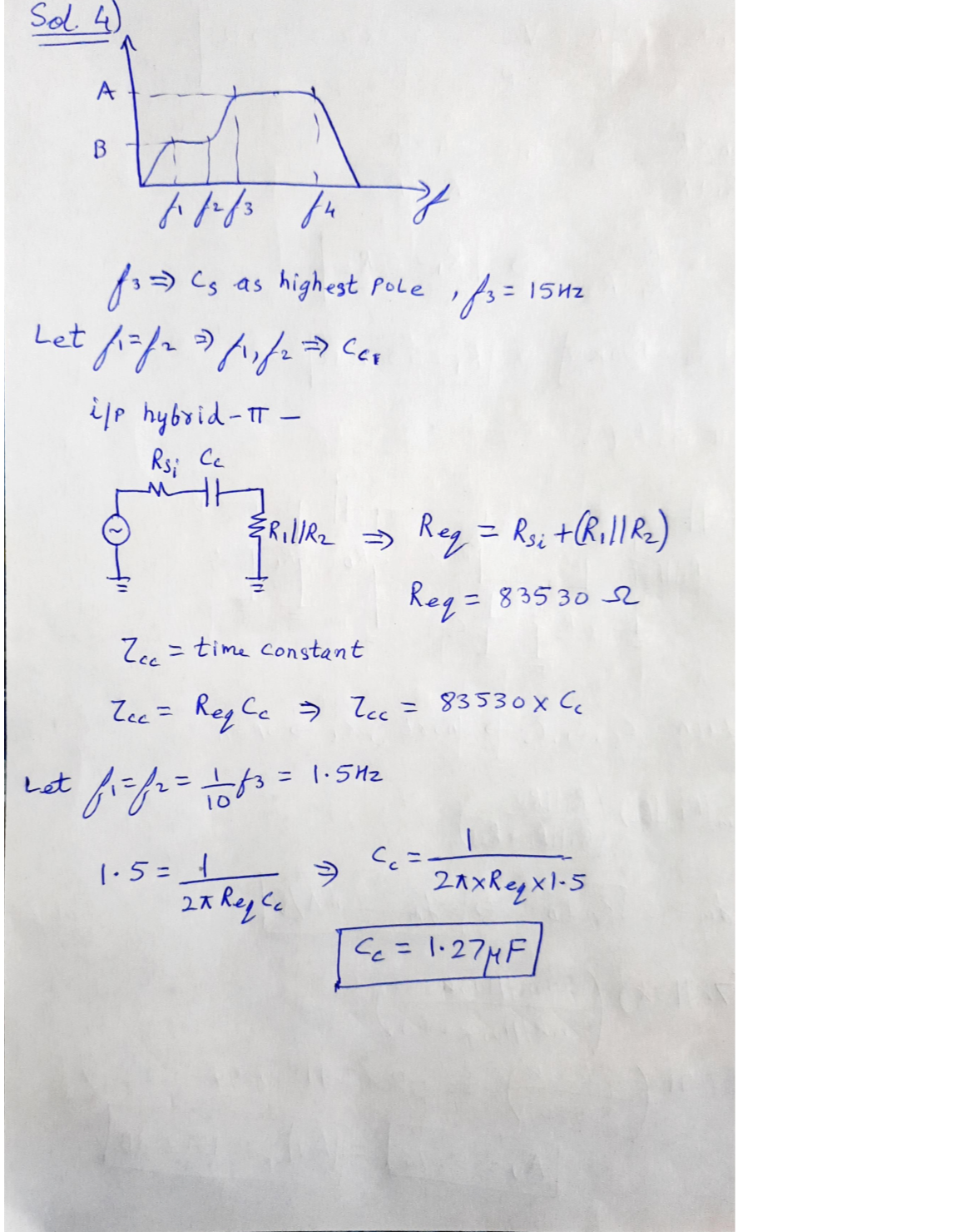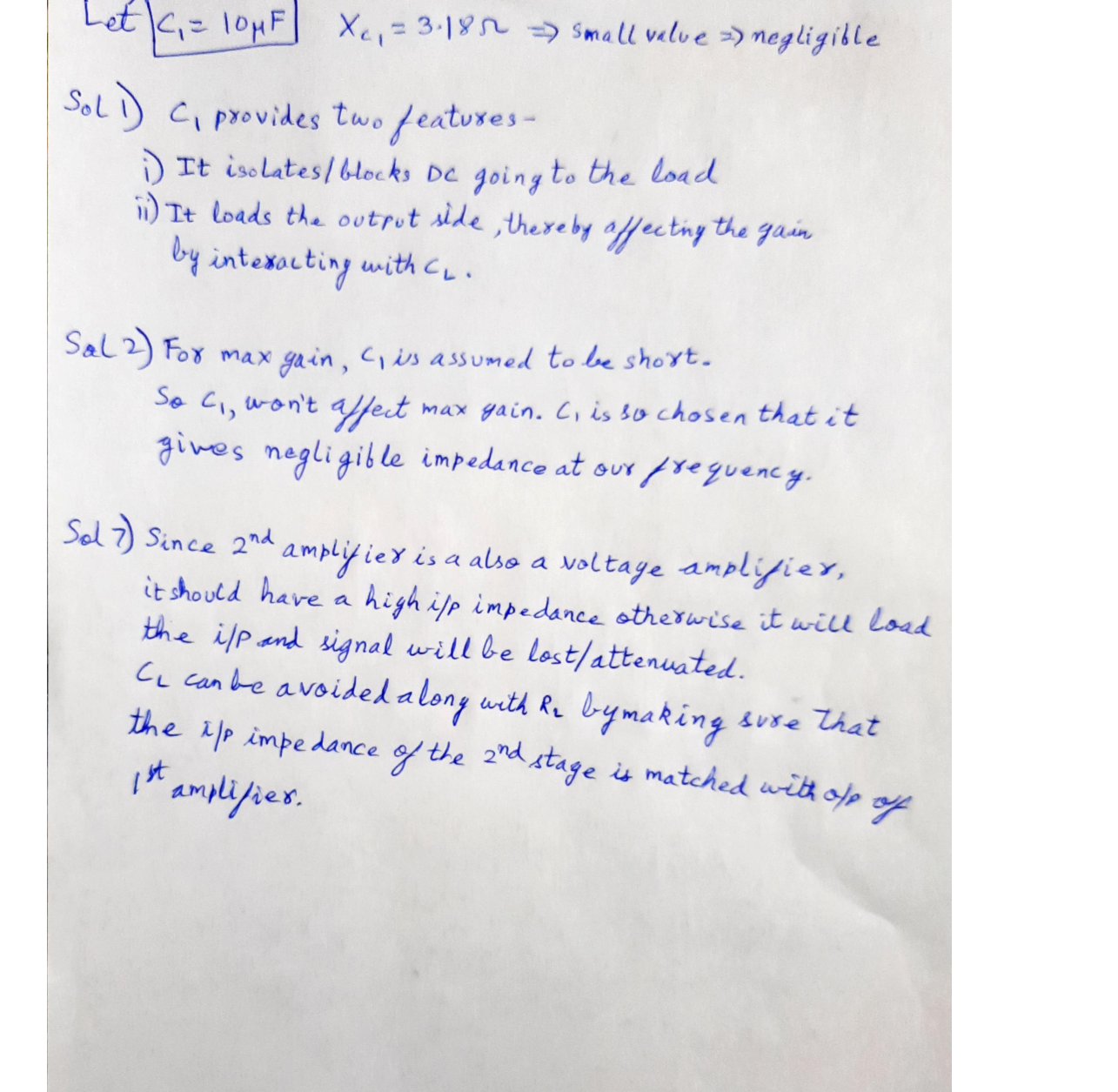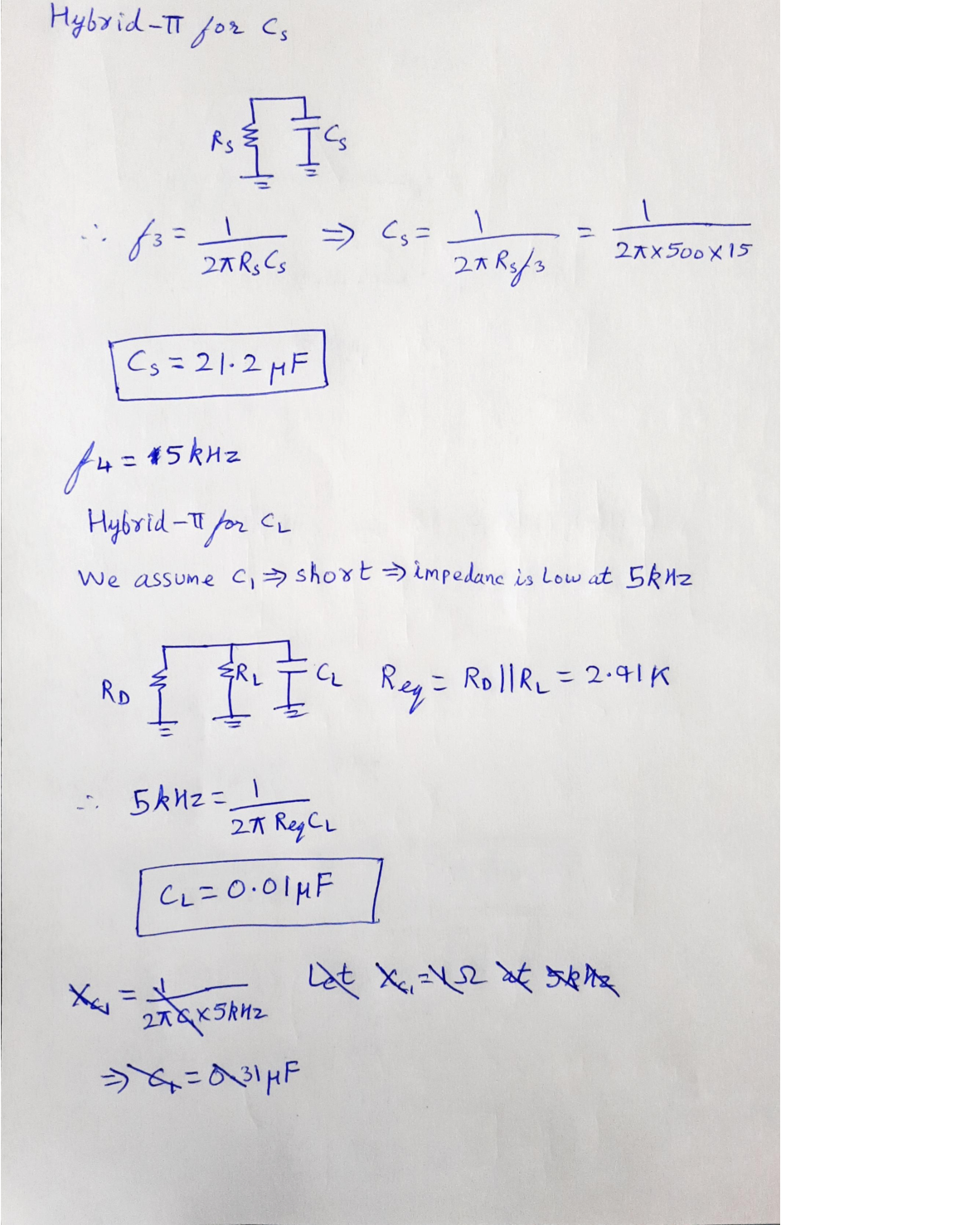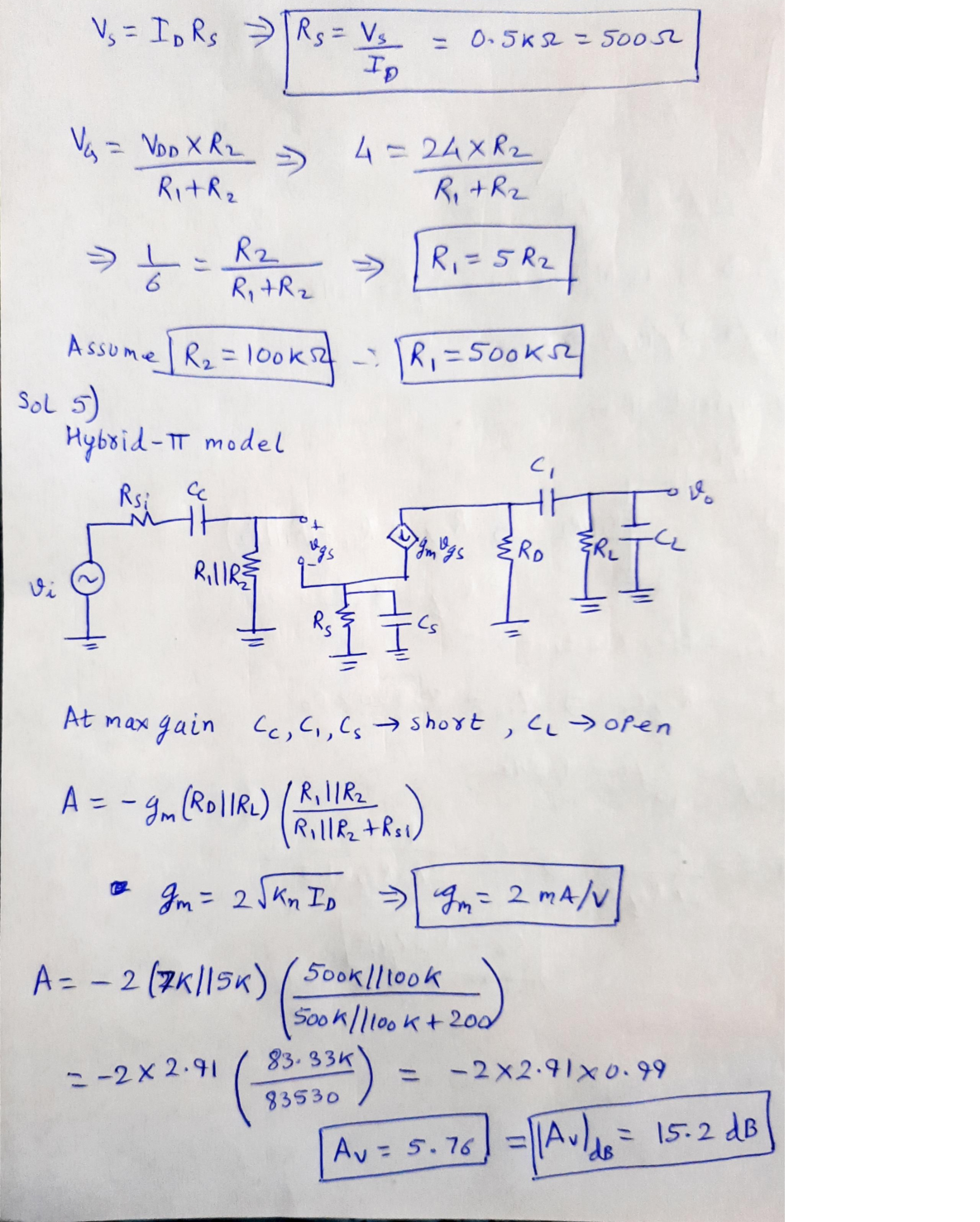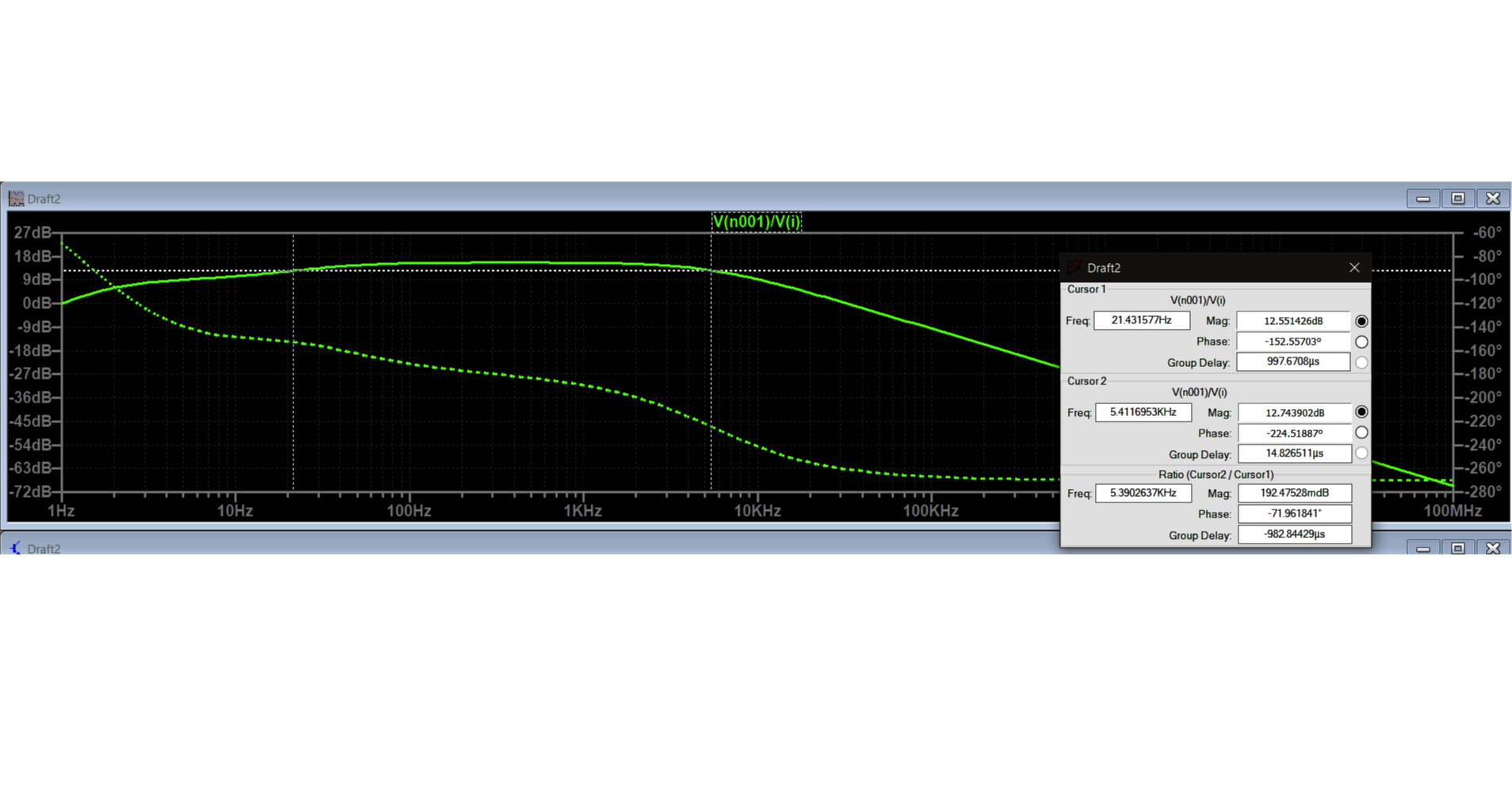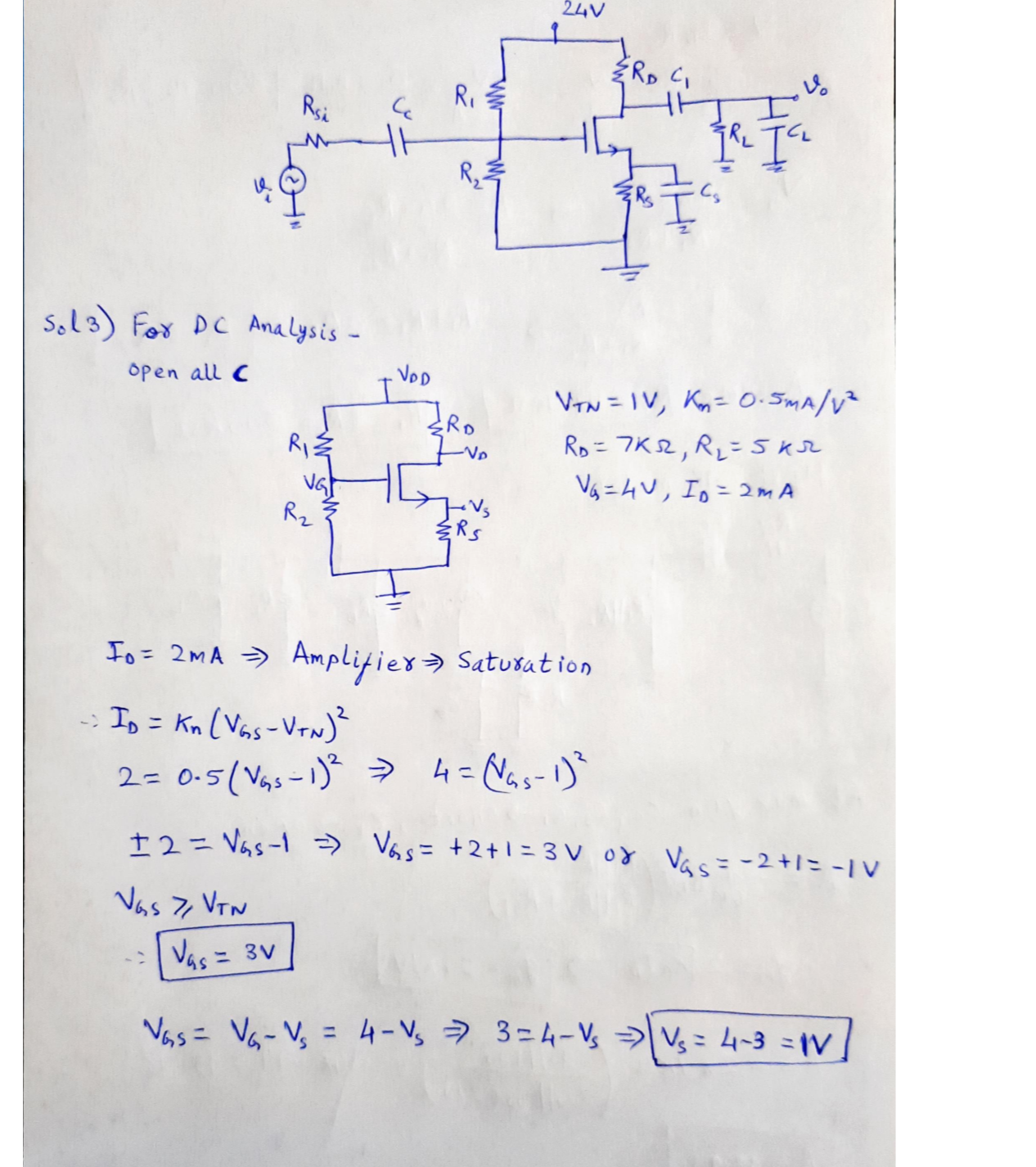### Question 45529Circuits

11) From your answer in (10), does the 4V 'DC' or steady state voltage impact the value of the displacement current, Ic.

### Question 45528Circuits

\text { 10) Assuming } I_{C}=C \frac{d V_{C}}{d t} \text {, find the displacement current for } V_{C}=e^{-\omega t}+4 V \text { and } V_{C}=
sin(wt) + 4 V.

### Question 45527Circuits

9) Based on your answer in (7), can you apply an arbitrarily large field or voltage across a dielectric? What is the name for this limitation and what is its value for Air and Glass (SiO2)?

### Question 45526Circuits

8) Remembering quantum tunneling from Chapter 3, what is happening to the probability of a tunneling event as the "thickness" of the barrier representing the dielectric decreases?

### Question 45525Circuits

7) What happens to the relative "thickness", or distance an electron must travel in a straight line across the dielectric, as you increase the voltage?

### Question 45524Circuits

6) Redraw the diagram in (5) with a small positive voltage on the right-hand side (no voltage or OVon the left-hand side).

### Question 45523Circuits

5) Draw the energy band diagram of a capacitor made of two aluminum plates with a glass dielectric. The capacitor should have zero applied voltage.

### Question 45522Circuits

\text { Given } C=\frac{\varepsilon A}{d}=\frac{q}{v} \text { and the units of } \varepsilon \text { are } F / m \text {, determine the equivalent units of the Farad (I.E. in }
terms of other available units).

### Question 45521Circuits

3) What is the result of increasing thickness of a dielectric (increasing d in the capacitor equation)while keeping the amount of charge on each plate the same? Think in terms of the capacitance and the voltage measured across the capacitor.

### Question 45520Circuits

2) Define the displacement current. Are charges physically flowing through the dielectric?

### Submit query